## Find the angle between v = 2i – j and w = 3i + 4jRound nearest tenth of a degree

Question

Find the angle between v = 2i – j and w = 3i + 4jRound nearest tenth of a degree

in progress 0
6 months 2021-08-13T16:53:44+00:00 2 Answers 1 views 0

## Answers ( )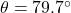Step-by-step explanation:

Given that,

v = 2i – j and w = 3i + 4j

We need to find the angle between v and w.

Magnitude of |v|,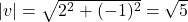Magnitude of |w|,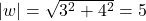The dot product of v and w,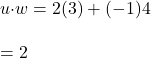The formula for the dot product is given by :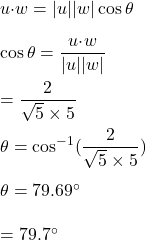So, the angle between u and v is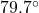.

The angle between two vectors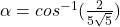∝ = 79.700°

Step-by-step explanation:

Explanation

Given V =  2i – j and w = 3 i + 4 j

Let ‘∝’ be the angle between the two vectors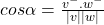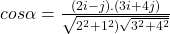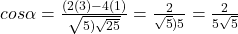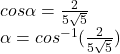The angle between two vectors

∝ = 79.77°